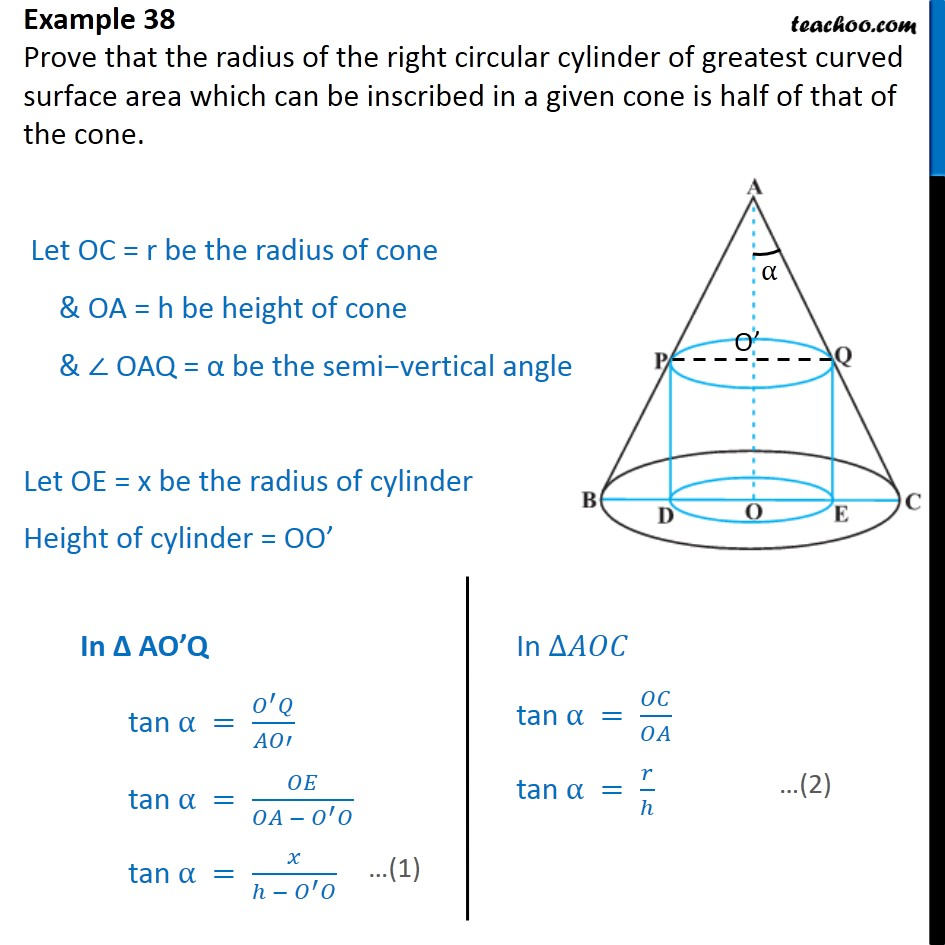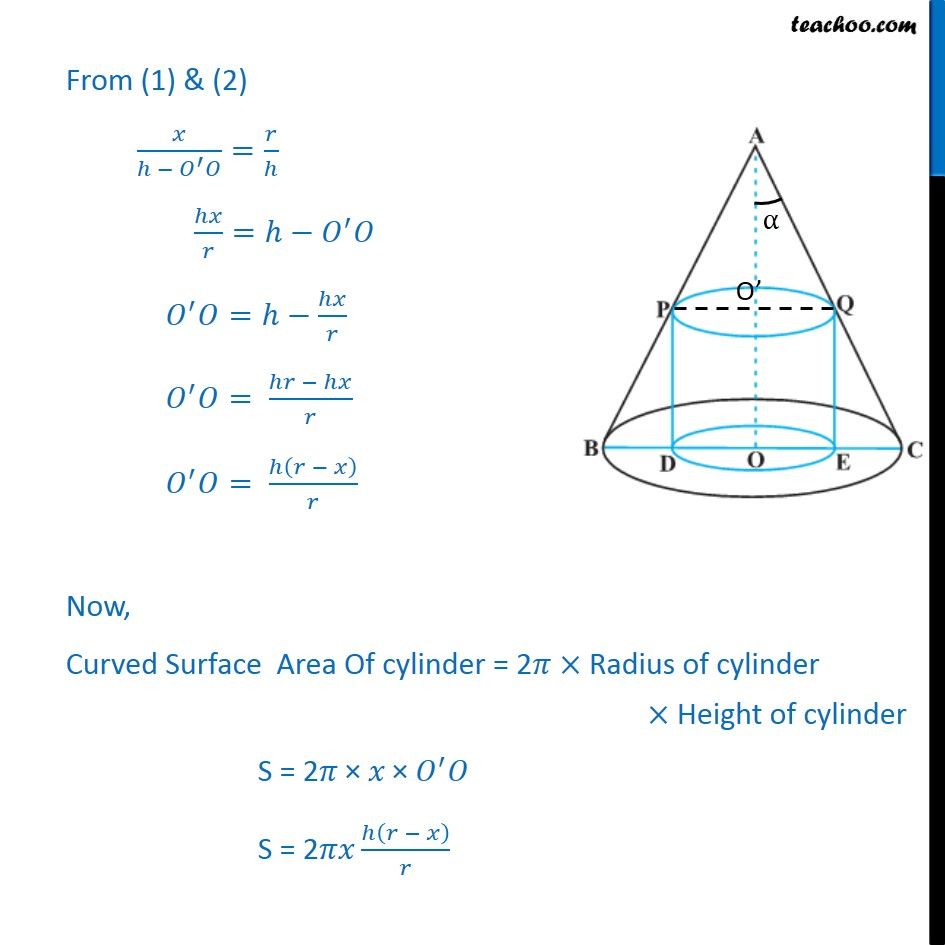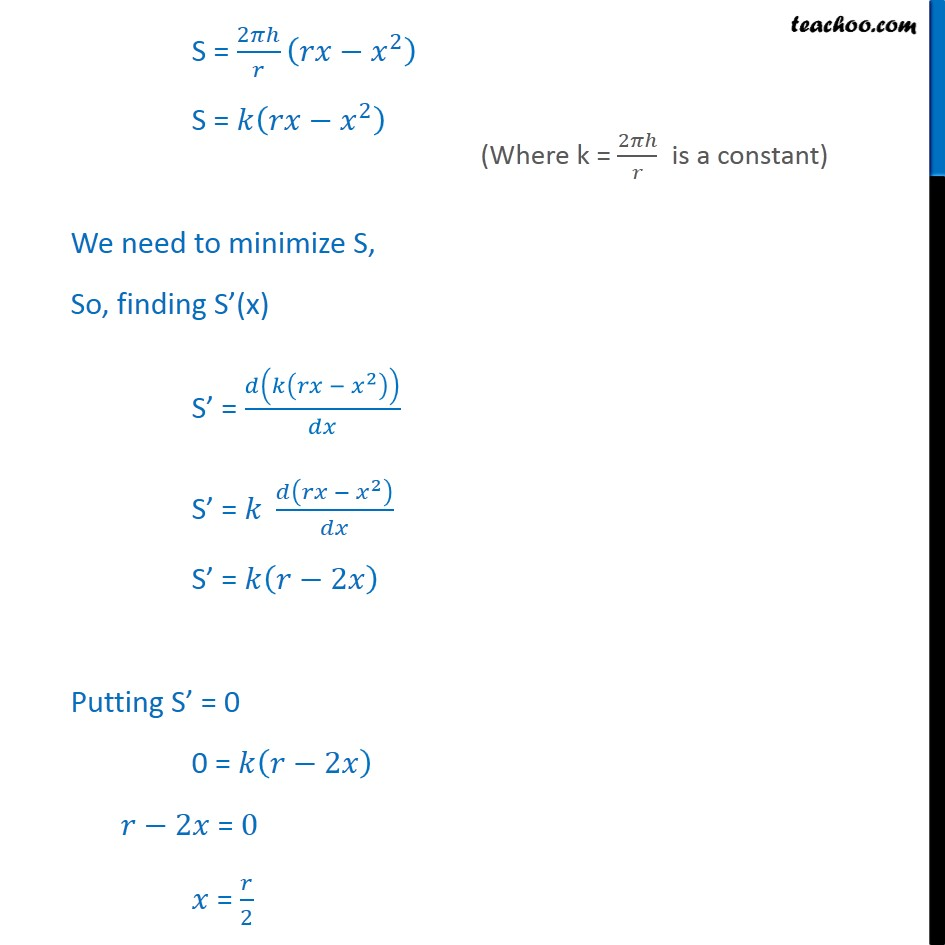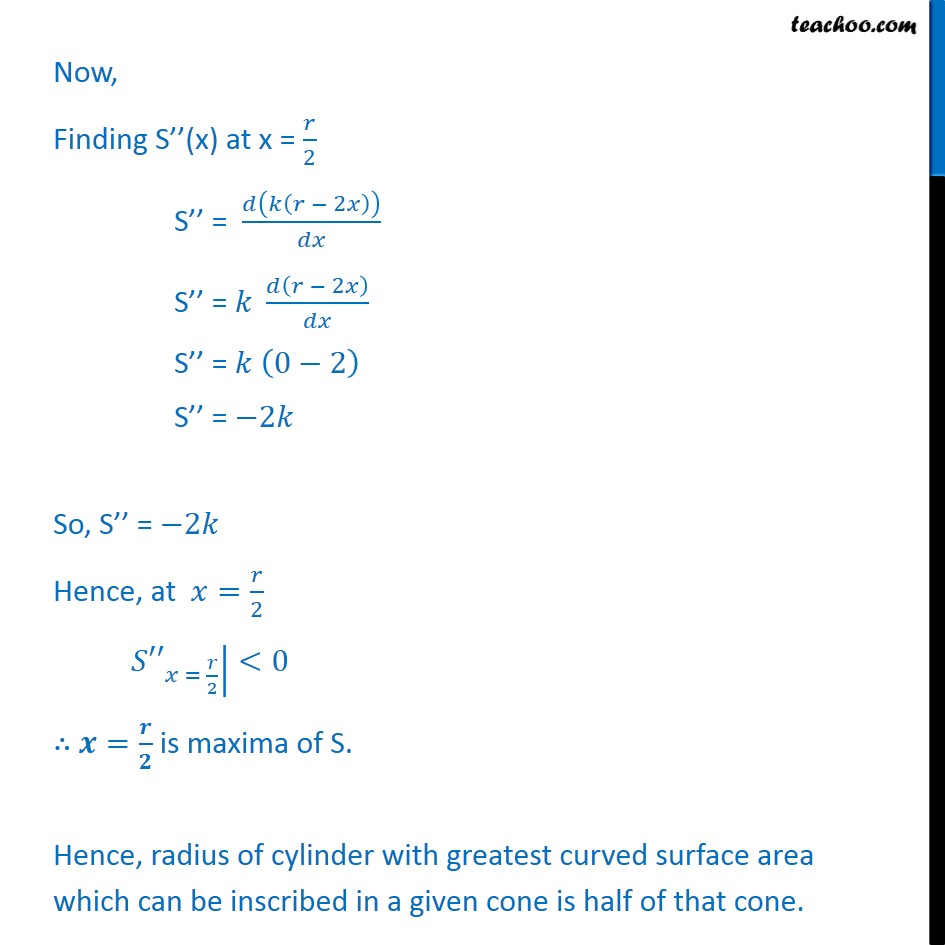1. Class 12
2. Important Question for exams Class 12
3. Chapter 6 Class 12 Application of Derivatives

Transcript

Example 38 Prove that the radius of the right circular cylinder of greatest curved surface area which can be inscribed in a given cone is half of that of the cone. Let OC = r be the radius of cone & OA = h be height of cone & OAQ = be the semi vertical angle of cone Let OE = x be the radius of cylinder Height of cylinder = OO From (1) & (2) = = = = = Now, Curved Surface Area Of cylinder = 2 Radius of cylinder Height of cylinder S = 2 S = 2 S = 2 2 S = 2 We need to minimize S, So, finding S (x) S = 2 S = 2 S = 2 Putting S = 0 0 = 2 2 = 0 = 2 Now, Finding S (x) at x = 2 S = 2 S = 2 S = 0 2 S = 2 So, S = 2 Hence, at = 2 = 2 <0 = is maxima of S. Hence, radius of cylinder with greatest curved surface area which can be inscribed in a given cone is half of that cone.

Chapter 6 Class 12 Application of Derivatives

Class 12
Important Question for exams Class 12